-=+=- -=+=- -=+=- -=+=- -=+=- -=+=- -=+=- -=+=- -=+=- -=+=- -=+=- -=+=- -=+=- -=+=- -=+=- -=+=- -=+=- -=+=- -=+=- -=+=- -=+=- -=+=- -=+=- -=+=- -=+=- -=+=- -=+=- -=+=- -=+=- -=+=- (c) WidthPadding Industries 1987 0|568|0 -=+=- -=+=- -=+=- -=+=- -=+=- -=+=- -=+=- -=+=- -=+=- -=+=- -=+=- -=+=- -=+=- -=+=- -=+=- -=+=- -=+=- -=+=- -=+=- -=+=- -=+=- -=+=- -=+=- -=+=- -=+=- -=+=- -=+=- -=+=- -=+=- -=+=-### therevillsgames

View Profile, Send Private Message
Recent Posts, Showcases, Articles, Code Snippets, Links, Wed.Workshop, Blog

### Latest Showcases

therevillsgames# Santa's Christmas Solitaire 2

20th December 2019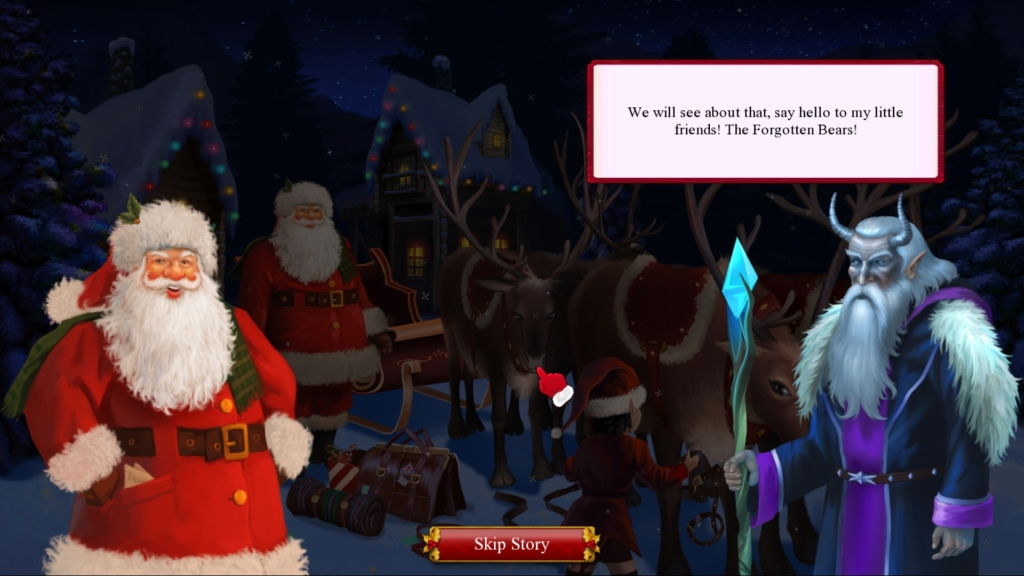Christmas + Solitaire = Santa's Christmas Solitaire 2

therevillsgames4th April 2019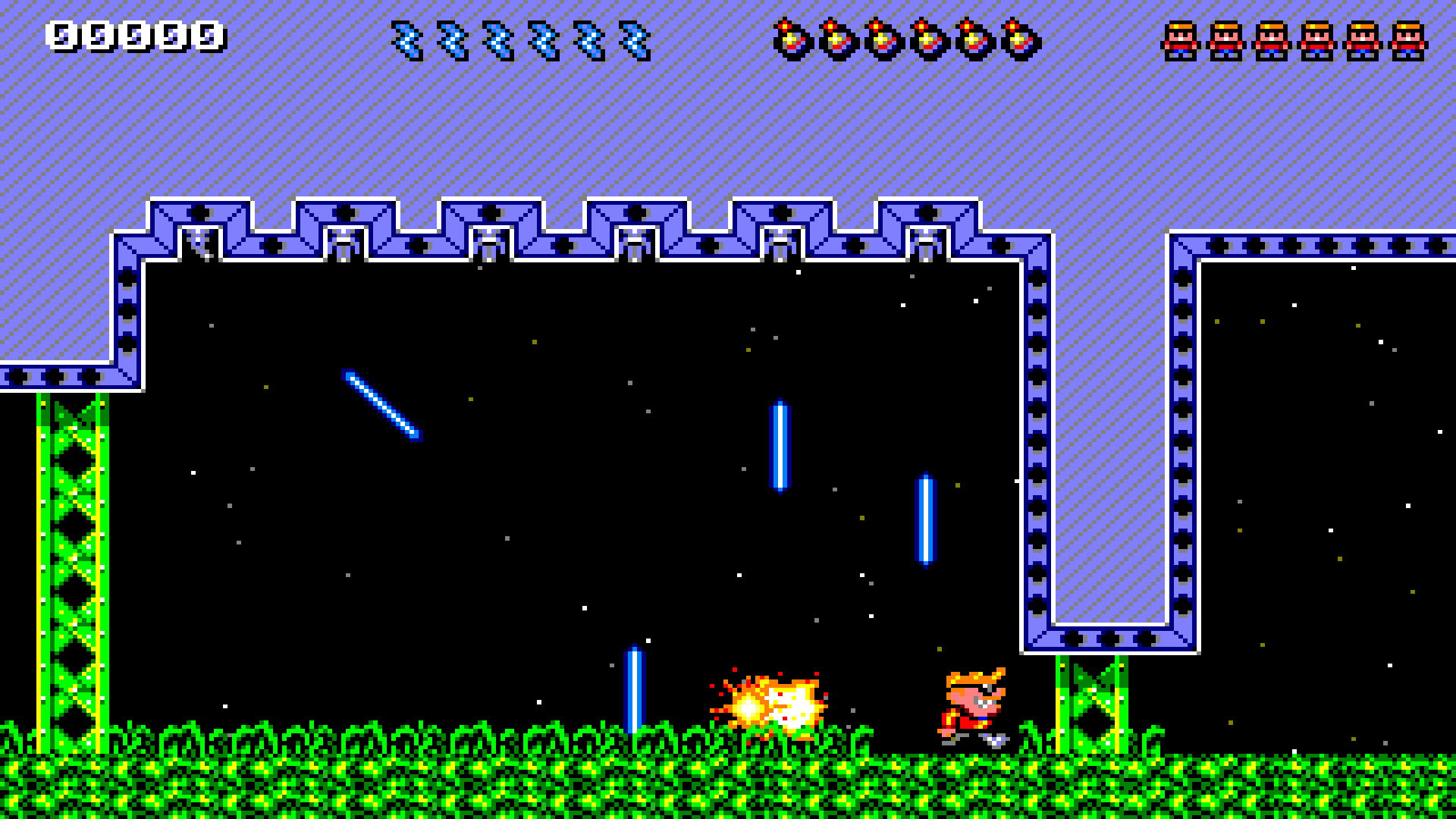When Rick dies, Jack steps up!

therevillsgames# Redshirt Fodder

30th June 2018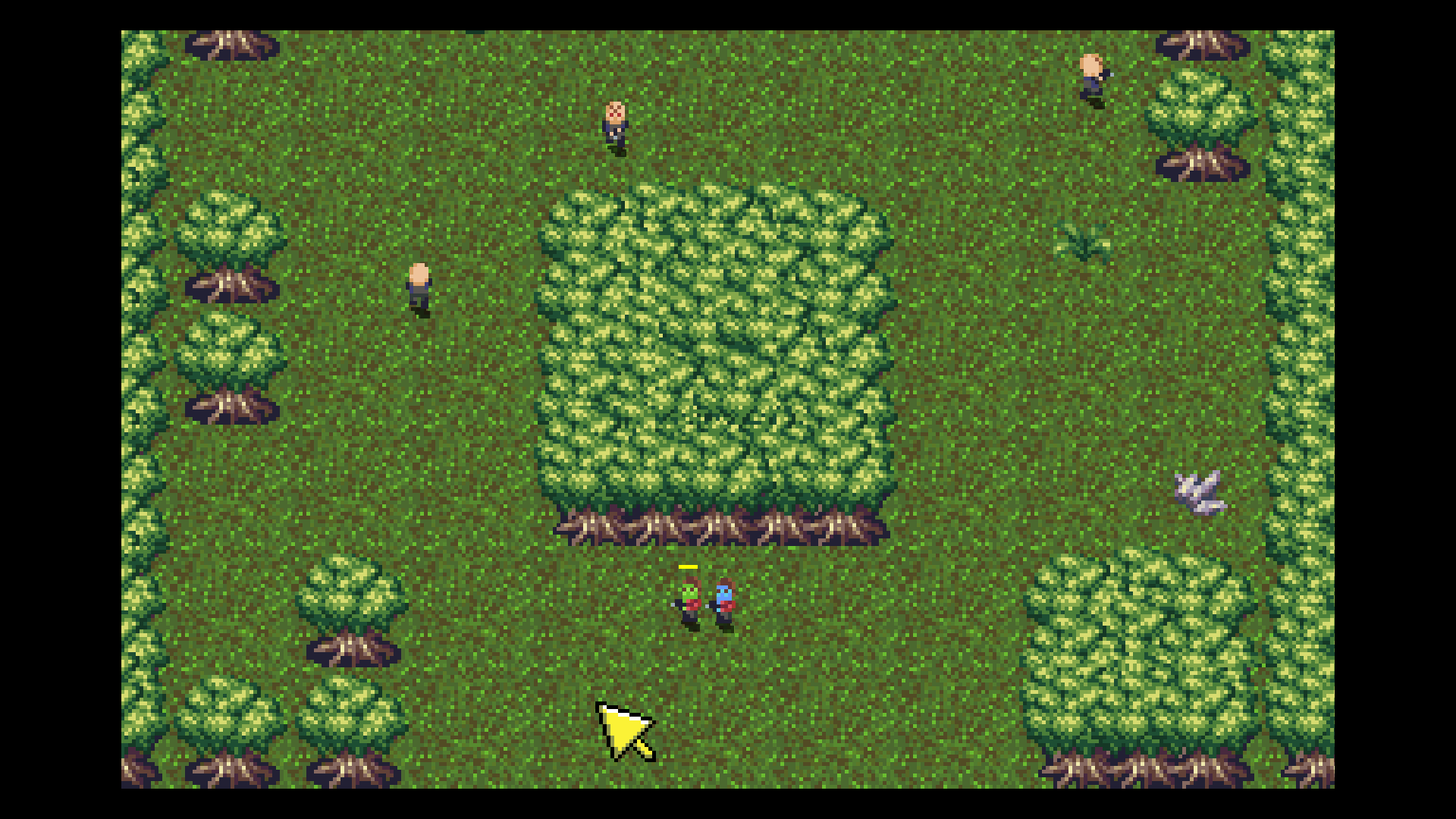To boldly die like no one has before...

therevillsgames# Get to Da Choppa!

20th January 2018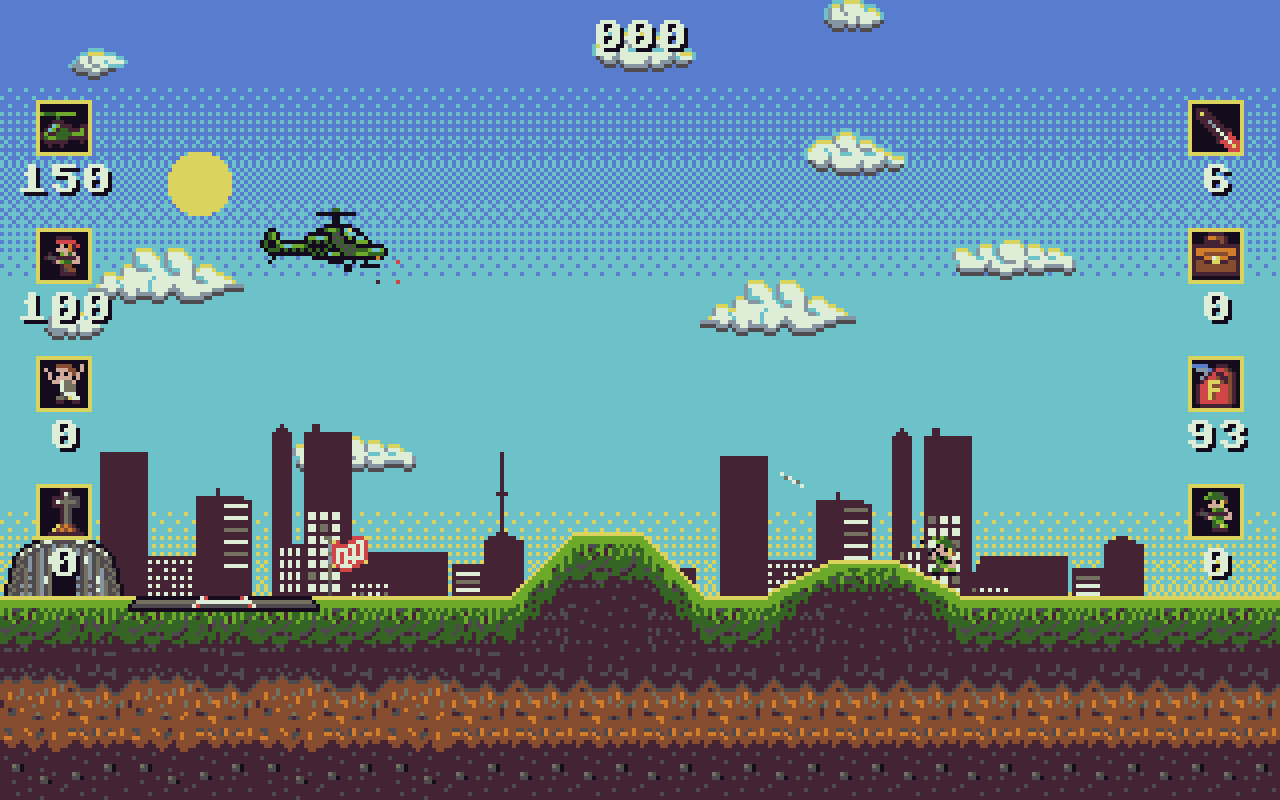[b]Get to Da Choppa[/b] is my entry for the 'Syntax Bomb' Retro Game competition 2017/18. 320x200 resolution and 16 colours.

Mostly inspired by the Amiga game: Apocalypse.

Developed in Monkey2!

[b]*** TOP SECRET ***[/b]

OPERATION: DA*snip*

therevillsgames# Home Run Tri Peaks Solitaire

17th November 2017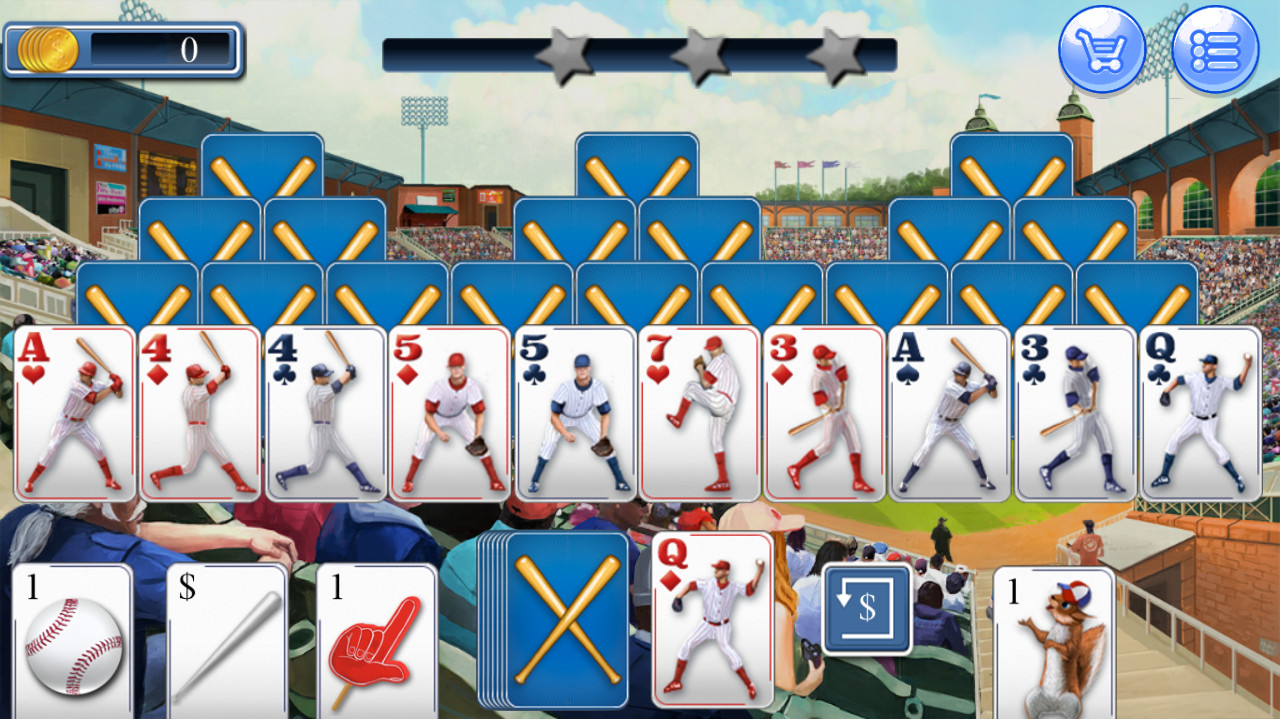Go hit a home run solitaire combo on Android!

therevillsgames# Insectoids!

23rd September 2017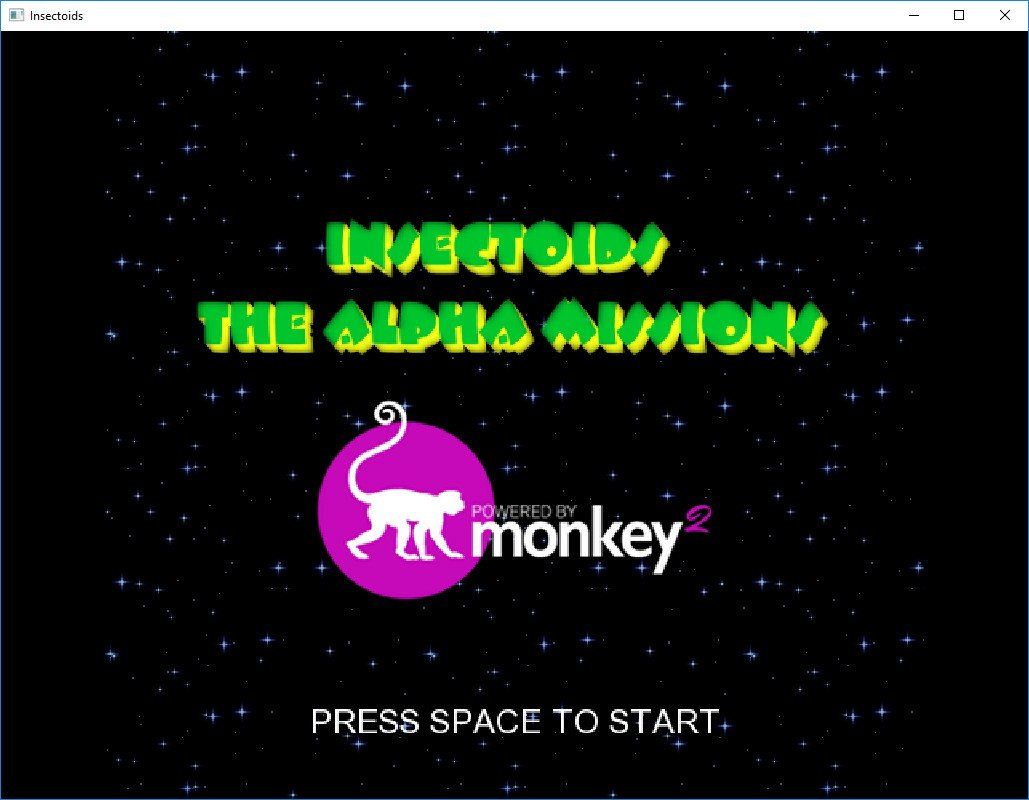Shoot the Bugs!